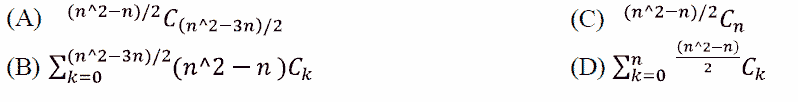Related Articles
GATE | GATE-CS-2004 | Question 90
• Difficulty Level : Hard
• Last Updated : 25 Sep, 2014

How many graphs on n labeled vertices exist which have at least (n2 – 3n)/2 edges ?(A) A
(B) B
(C) C
(D) D

Answer: (D)

Explanation:

Let there be total number of edges of a graph to be formed be e and total number of vertices be n.

So the total number of ways a graph can be formed having exactly e edges and v vertices is given by the total number of ways we can select e edges among v edges

i. e.

C (v, e)

Now, here e = (n2 – 3n)/2 and v = n(n-1)/2 (maximum number of edges in a simple graph).

So to select a edge we can do that in C (v, (n2 – 3n)/2) ways.

Since minimum no. of edges to be selected for a graph are (n2 – 3n)/2.

So total number of graphs possible will be :

C(v, e) + C(v, e+1) + C(v, e+2) +……………+ C(v, v).

C(v, v-e) + C(v, v-(e+1)) + C(v, v-(e+2)) +……………+ C(v, v-v).

Since v – e = n(n-1)/2 – (n2 – 3n)/2 = n

Therefore

C(v, n) + C(v, n-1) + C(v, n-2) +……………+ C(v, 0).

Solving this we will get

This solution is contributed by Namita Singh.My Personal Notes arrow_drop_up